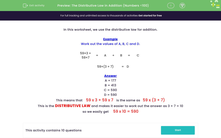# The Distributive Law in Addition (Numbers <100)

In this worksheet, students use the distributive law to work out a calculation.Key stage:  KS 3

Curriculum topic:   Number

Curriculum subtopic:   Use Relationships Between Operations

Difficulty level:#### Worksheet Overview

In this worksheet, we use the distributive law for addition.

Example

Work out the values of A, B, C and D.

59×3 + 59×7 =
 A
+
 B
=
 C

59×(3 + 7) =
 D

A = 177
B = 413
C = 590
D = 590

This means that    59 x 3 + 59 x 7   is the same as   59 x (3 + 7)

This is the DISTRIBUTIVE LAW and makes it easier to work out the answer as 3 + 7 = 10

so we easily get     59 x 10 = 590

### What is EdPlace?

We're your National Curriculum aligned online education content provider helping each child succeed in English, maths and science from year 1 to GCSE. With an EdPlace account you’ll be able to track and measure progress, helping each child achieve their best. We build confidence and attainment by personalising each child’s learning at a level that suits them.

Get started